# Spectral Graph Theory Sample

The spectral graph theory studies the properties of graphs by means of an analysis of eigenvalues / eigenvectors / of characteristic polynomials of matrices that are connected with graphs (conjugacy matrix, Laplace matrix, unsigned Laplace matrix, etc.). The theory was actively developed in the 1970s, but now finds application in the analysis of graphs of social networks. The theory is related to the detection of communities and the partitioning of graphs. The sample that you will find below will be devoted to theoretical aspects (even proofs), and will be useful for applicants to understand why certain methods of generating features (based on spectra) are working.

In the sample, we present the introduction to spectral graph theory. This sample allows you to deal with your assignment much easier. The point is that you can use the sample if you need to deal with the same topic, but don’t present it as your own assignment. So, check out our sample if you need to do a task on spectral graph theory.

Some Results From Spectral Graph Theory

In this short overview, we briefly examine the connection between a graph and eigenvalues along with eigenvectors of its adjacency matrix, which together are usually referred to as the spectrum of a matrix. At first glance, computing eigenvalues of the adjacency matrix may not seem to be a promising idea in terms of getting a somewhat useful information about a graph, a mathematical object that lives in a completely different dimension. But such a conclusion is quite unfair. It turns out that the spectrum of the adjacency matrix can provide us with deep insight into some of the key characteristics of a graph in an unexpectedly concise and elegant manner. Moreover, there exists a separate well-established discipline called spectral graph theory, which is completely dedicated to the investigation of the spectrum of various matrices associated with graphs.

To establish this seemingly vague connection lets first recall the definitions of a graph, its adjacency matrix, and the spectrum of a matrix. Graphis an ordered pair (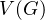,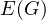) consisting of a setof vertices and a set, disjointed from, of edges, together with the incidence function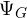that associates with each edge ofan unordered pair of vertices of. Then, the adjacency matrix ofis the matrix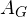:= (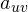), whereis the number of edges joining vertices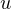and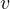, each loop counting as two edges . Finally, given matrix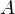, an eigenvalue of a matrix is a number such that for some non-zero vectorwe have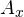=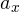. The vectorsatisfies this identity called eigenvector corresponding to the eigenvalue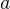.

Since the adjacency matrix of a graph is a symmetric matrix, the number of its eigenvalues is exactly the number of vertices in the graph. When studying the spectrum of graphs, researchers are usually most concerned about how eigenvalues are related to each other, the biggest and the smallest ones, or how large the gap is between two subsequent eigenvalues. The first interesting fact to notice is that the largest eigenvalue of the adjacency matrix is at least the average degree of a vertex of the graph and at most the maximum degree of a vertex of the graph . Also, such an important characteristic of a graph as connectedness implies that the two largest eigenvalues are distinct . This fact gives us a fast and elegant way to check if the graph is disconnected. A lot of important results can be obtained from the Laplacian matrix of a graph, which can be computed by adding some information about vertex degree to the adjacency matrix. It turns out that a graph is only bipartite if the largest eigenvalue of its Laplacian matrix has the same magnitude as the smallest one, but their signs are opposite . Furthermore, the eigenvectors of the Laplacian matrix can help us to get a nice graph drawing .

Probably one of the most famous applications of the spectral graph theory is the PageRank algorithm invented in 1996 by the founders of Google, Sergey Brin and Larry Page. Consider a graph representing a network of web pages. We first compute its adjacency matrix, and after some modifications get the so-called Google Matrix . We then compute its largest eigenvalue. It turns out that the eigenvector corresponding to this eigenvalue describes the ranking of the web pages in the network, and namely its coordinates give us a probability distribution of ending up at the particular web page after a sufficient amount of surfing time. The PageRank algorithm was one of the key factors of Google’s tremendous success and it still remains as one of Google’s key search engine components.

By any means, we did not try to provide a comprehensive overview of the spectral graph theory. We barely scratched the surface of this important area of modern mathematics. There are lots of other deep and surprising results, and this text is just an invitation to learn more!